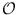Next: Depth First Search (flexible) Up: Graphs and Iterators Previous: Node Attribute Accessors (   Contents   Index

# Breadth First Search (flexible) ( GIT_BFS )

Definition

An instance algorithm of class GIT_BFS< OutAdjIt, Queuetype, Mark > is an implementation of an algorithm that traverses a graph in a breadth first order. The queue used for the search must be provided by the caller and contains the source(s) of the search.

• If the queue is only modified by appending the iterator representing the source node onto the queue, a normal breadth first search beginning at the node of the graph is performed.

• It is possible to initialize the queue with several iterators that represent different roots of breadth first trees.

• By modifying the queue while running the algorithm the behaviour of the algorithm can be changed.

• After the algorithm performed a breadth first search, one may append another iterator onto the queue to restart the algorithm.

Iterator version: There is an iterator version of this algorithm: BFS_It. Usage is similar to that of node iterators without the ability to go backward in the sequence.

#include < LEDA/graph/graph_iterator.h >

Creation

 GIT_BFS< OutAdjIt, Queuetype, Mark > algorithm(const Queuetype& q, Mark& ma) creates an instance algorithm of this class bound to the Queue q and data accessor ma.

Preconditions:

• Queuetype is a queue parameterized with items of type OutAdjIt.
• q contains the sources of the traversal (for each source node an adjacency iterator referring to it) and
• ma is a data accessor that provides read and write access to a boolean value for each node (accessed through iterators). This value is assumed to be freely usable by algorithm.

 GIT_BFS< OutAdjIt, Queuetype, Mark > algorithm(const Queuetype& q, Mark& ma, const OutAdjIt& ai) creates an instance algorithm of this class bound to the queue q, data accessor ma and the adjacency iterator ai representing the source node of the breadth first traversal.

Operations

 void algorithm.next() Performs one iteration of the core loop of the algorithm. OutAdjIt algorithm.current() returns the current'' iterator. void algorithm.finish_algo() executes the algorithm until finished() is true, i.e. exactly if the Queue is empty. bool algorithm.finished() returns true if the internal Queue is empty. Queuetype& algorithm.get_queue() gives direct access to internal Queue.

Example

This example shows how to implement an algorithmic iterator for breadth first search:

class BFS_It {
node_array<da>      _handler;
node_array_da<bool> _mark;
public:
BFS_It(graph& G) :
_mark(_handler), _search(_q,_mark)
{
_search.get_queue().clear();
_search.get_queue().append(_source);
}
bool valid() const { return !_search.finished();  }
node get_node() const { return _search.current().get_node(); }
BFS_It& operator++() {
_search.next(); return *this; }
};


With this iterator you can easily iterate through a graph in breadth first fashion :

  graph G;
BFS_It it(G);
while (it.valid()) {
// do something reasonable with 'it.get_node()'
++it;
}


Implementation

Each operation requires constant time. Therefore, a normal breadth-first search needs(m + n) time.Next: Depth First Search (flexible) Up: Graphs and Iterators Previous: Node Attribute Accessors (   Contents   Index